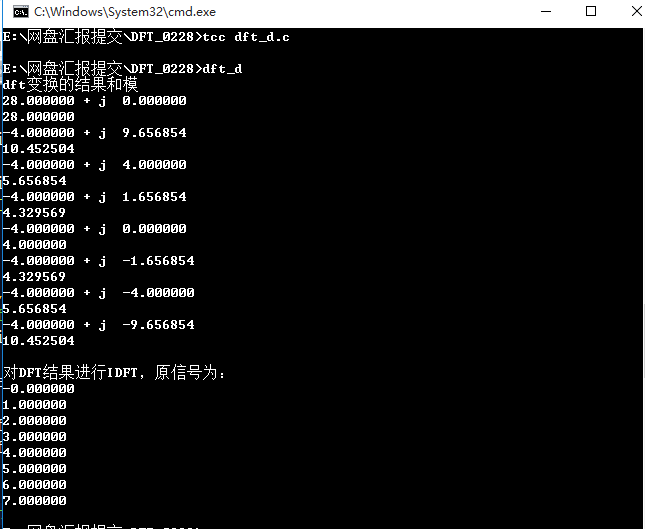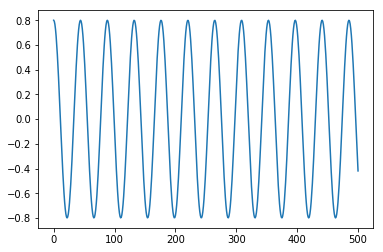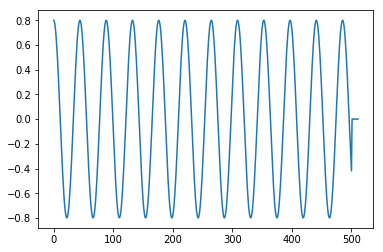• 该文件包括用于 DFT 和 IDFT 的 matlab 代码matlab
• 使用 Direct 和 FFT 方法计算 DFT、IDFTmatlab
• 无需使用内置函数即可找到离散信号的 DFT 和 IDFT。它是快速傅立叶变换 (FFT) 的替代算法matlab
• ## DFT与IDFT

万次阅读 2019-02-28 20:38:01
DFT与IDFT 一.方法简介 序列x(n)(n=0,1,…N-1)的DFT定义为 X（k）=∑n=0N−1x(n)e−j2πnkN X（k）=\sum_{n=0}^{N-1}x(n)e^{-j\frac{2\pi nk}{N}} X（k）=n=0∑N−1​x(n)e−jN2πnk​ 设x(n)=a(n)+jb(n),X(k)...
DFT与IDFT
一.方法简介
序列x(n)(n=0,1,…N-1)的DFT定义为
$X（k）=\sum_{n=0}^{N-1}x(n)e^{-j\frac{2\pi nk}{N}}$
$设x(n)=a(n)+jb(n),\quad X(k)=A(k)+jB(K),\quad Q=2\pi/N$
则上式变为：
$A（k）+jB(k)=\sum_{n=0}^{N-1}[a(n)+jb(n)][\cos(Qnk)-j\sin(Qnk)]$
即
$A（k）=\sum_{n=0}^{N-1}[a(n)\cos(Qnk)+b(n)\sin(Qnk)]$
$B(k)=\sum_{n=0}^{N-1}[b(n)\cos(Qnk)-a(n)\sin(Qnk)]$
序列X（k）的IDFT定义为：
$x(n)=\frac{1}{N}\sum_{k=0}^{N-1}X(k)W_{N}^{-nk},\quad n=0,1,...N-1$
$它与DFT的区别在于将W_{N}改变为改变为W_{N}^{-1},并多了一个除以N 的运算，公式如下$
$a(n)=\frac{1}{N}\sum_{k=0}^{N-1}[A(k)\cos(Qnk)-B(k)\sin(Qnk)]$
$b(n)=\frac{1}{N}\sum_{k=0}^{N-1}[B(k)\cos(Qnk)+A(k)\sin(Qnk]$
二.编程实现
考滤到DFT和IDFT算法过程中有部分相似，可以把它们合成到一个算法。
DFT.c
/*
x-存放要变换数据的实部
y-存放要变换数据的虚部
a-存放变换结果的实部
b-存放变换结果的虚部
n-数据长度
sign-为1时执行DFT，为-1时执行IDFT
*/
#include "math.h"
void dft(x,y,a,b,n,sign)
int n, sign;
double x[],y[],a[],b[];
{
int i,k;
double c,d,q,w,s;
q = 6.28318530718/n;
for (k=0;k<n;k++)
{
w=k*q;
a[k]=b[k]=0.0;
for(i=0;i<n;i++)
{
d=i*w;
c=cos(d);
s=sin(d)*sign;
a[k]+=c*x[i] + s*y[i];
b[k]+=c*y[i] - s*x[i];
}
}
if(sign == -1)
{
c=1.0/n;
for (k=0;k<n;k++)
{
a[k]=c*a[k];
b[k]=c*b[k];
}
}
}

下面验证此算法，对X(n)=(0,1,2,3,4,5,6,7)，做DFT和IDFT算法
dft_d.c
#include "stdio.h"
#include "math.h"
#include "dft.c"
#define N 4
static double  x[N],y[N],a[N],b[N],c[N];
main(){
int k;
int i=0;
for(i=0; i<N; i++)
{
x[i]=i;
y[i]=0;

}
dft(x,y,a,b,N,1);	//DFT变换
for(i=0; i<N; i++)
{
c[i]=sqrt(a[i]*a[i]+b[i]*b[i]);	//算出模
printf("%lf + j  %lf \n",a[i],b[i]);//输出变换后结果
printf("%lf \n",c[i]); //输出模值
printf("\n");
}
dft(a,b,x,y,N,-1); //IDFT变换
for(i=0; i<N; i++)
{
printf("%lf \n",x[i]); //输出x(n)的实部
}

}

运行结果：展开全文• matlab的idft代码Matlab的 用于时域和频域采样的Matlab代码用于使用DFT和IDFT进行线性和圆形卷积
• ## DFT和IDFT分析

千次阅读 2019-08-26 20:45:00
DFT和IDFT分析DFT和IDFT的意义DFT的定义DFT的矩阵分析 DFT和IDFT的意义 DFT：离散傅里叶变换 离散傅里叶变换可以将连续的频谱转化成离散的频谱去计算，这样就易于计算机编程实现傅里叶变换的计算。FFT算法的出现...
DFT和IDFT分析DFT和IDFT的意义定义DFT的定义DFT的定义DFT的矩阵分析DFT在matlab相关的代码分析
DFT和IDFT的意义
DFT：离散傅里叶变换
离散傅里叶变换可以将连续的频谱转化成离散的频谱去计算，这样就易于计算机编程实现傅里叶变换的计算。FFT算法的出现，使得DFT的计算速度更快。
定义
DFT的定义
设 $x(n)$ 是一个长度为$M$的有限长序列，则定义$x(n)$的$N$点离散傅里叶变换为
$X(k) = DFT[x(n)] = \sum\limits_{n = 0}^{N - 1} {x(n)W_N^{kn}} \ \ \ \ \ \ \ (k=0, 1, ..., N-1)$
注意：$x(n)$的离散傅里叶变换结果与变换区间长度$N$的取值有关。
DFT的定义
$X(k)$的离散傅里叶逆变换（Inverse Discrete Fourier Transform, IDFT）为
$x(n) = IDFT[X(k)]=\frac{1}{N} \sum\limits_{n = 0}^{N - 1} {X(k)W_N^{-kn}} \ \ \ \ \ \ \ (n=0, 1, ..., N-1)$
式中， $W_{N}=e^{-j\frac{2\pi}{N}}$, $N$称为DFT变换区间长度，$N \ge M$
DFT的矩阵分析
由DFT的数学表达式可以看出：

对于每一个$X(k)$的计算，为两个向量的内积形式

对于$k=0, 1, ..., N-1$,则$X(k)$的计算可以看做向量$x(n)$和一个矩阵的乘积，我们一般讲这个矩阵称作DFT矩阵。$X(k) = DFT[x(n)] = \sum\limits_{n = 0}^{N - 1} {x(n)W_N^{kn}} = x(n)*P \ \ \ \ \ \ \ (k=0, 1, ..., N-1)$        $P= \left[ \begin{array}{l} W_N^{0*0}\ \ W_N^{0*1} \ \ ...W_N^{0*n}\\ W_N^{1*0}\ \ W_N^{1*1} \ \ ...W_N^{1*n}\\ ... \\ W_N^{k*0}\ \ W_N^{k*1} \ \ ...W_N^{k*n}\\ \end{array} \right]$

同理，对于IDFT的运算，同样会有一个对应的IDFT矩阵，且和DFT矩阵有如下的关系: $x(n) = IDFT[X(k)]=\frac{1}{N} \sum\limits_{n = 0}^{N - 1} {X(k)W_N^{-kn}} =\frac{1}{N} X(k)*Q \\ (k=0, 1, ..., N-1)$        $Q= \left[ \begin{array}{l} W_N^{-0*0}\ \ W_N^{-0*1} \ \ ...W_N^{-0*n}\\ W_N^{-1*0}\ \ W_N^{-1*1} \ \ ...W_N^{-1*n}\\ ... \\ W_N^{-k*0}\ \ W_N^{-k*1} \ \ ...W_N^{-k*n}\\ \end{array} \right]$

对$P$和$Q$的形式进行分析，则可得到如下结论:
$Q = P^{*}$
其中，$P$是$DFT$矩阵，$Q$是$IDFT$矩阵，且$P$和$Q$均为对称阵。
$P$和$Q$的第一行和第一列全为1。

将$P$和$Q$应用于DFT和IDFT中，则有
$X(k) = DFT[x(n)] = x(n)*P \\ x(n) = IDFT[X(k)]=\frac{1}{N} X(k)*Q =\frac{1}{N} x(n)*P*Q \\ \frac{1}{N} x(n)*P*Q = I_{N}$
$P*Q = N * I_{N}$
说明$DFT$矩阵$P$和$IDFT$矩阵$Q$均为正交矩阵（$A*A^{H}=E$）。
这两个矩阵的每一行（列）都是有个基，在N个方向上都有不同的基向量。
因此，$DFT$和$IDFT$都是一种正交变换。

DFT在matlab
对于matlab中的$DFT$正变换，可使用内置函数dftmtx(n)，而对于$IDFT$的矩阵，可以通过$\frac{1}{N}*dftmtx(N)^{*}$来得到。
相关的代码分析
%% Prepare
clc;
close all;
clear;

%% Define the signal
N = 8;
x = [0:N-1];

%% Generate the vector of n and k
N = length(x);
n = [0:N-1];
k = [0:N-1];

%% Generate the twist-factor W
imag_unit = sqrt(-1);
W = exp(-1*imag_unit*2*pi/N);

%% Generate P matrix
P = W.^(n'*k);

%% DFT transformation
X_P = x*P;
X_fft = fft(x);
X_err = norm(X_P - X_fft)

%% Generate Q matrix
Q = conj(P);

% IDFT transformation
x_Q = 1/N * X_P * Q;
x_ifft = ifft(X_fft);
x_err = norm(x_Q - x_ifft)

%% Test: P*Q == N*I_n
I_n = eye(N);
left = P*Q;
right = N*I_n;
err = norm(left - right)

%% Generate P and Q using dftmtx
P_dftmtx = dftmtx(N);
P_err = norm(P_dftmtx - P)

Q_dftmtx = conj(dftmtx(N));
Q_err = norm(Q_dftmtx - Q)




展开全文FFT DFT矩阵
• 图像的DFT与IDFT，对abs的图像系数一分为四，分别进行IDFTmatlab
• ## IDFT的python实现

千次阅读 2018-11-07 16:19:12
IDFT IDFT(Inverse Discrete Fourier Transform), 傅里叶逆变换，可以将频域信号转换到时域中, 它的公式非常简单： x[n]=1N∑k=0N−1X[k]ej2πkn/N x[n] = \frac{1}{N} \sum_{k=0}^{N-1} X[k] e^{j2\pi kn/N} x[n]=...
IDFT
IDFT(Inverse Discrete Fourier Transform), 傅里叶逆变换，可以将频域信号转换到时域中, 它的公式非常简单：
$x[n] = \frac{1}{N} \sum_{k=0}^{N-1} X[k] e^{j2\pi kn/N}$
$X[k]$：离散频率下标为k时的频率大小
$x[n]$: 离散时域信号序列
$N$: 信号序列的长度，也就是采样的个数
对比我们之前讲过的DFT，两者公式类似，但是注意在DFT中指数带负号，而IDFT中不带
从矩阵的角度看IDFT
DFT的矩阵表示
讲IDFT之前，我们先复习DFT的矩阵表示形式：
$\begin{bmatrix} s_0^0 & s_0^1 & \cdots & s_0^{N-1} \\ \vdots & \vdots & \vdots & \vdots\\ s_k^0 & s_k^1 & \cdots & s_k^{N-1} \\ \vdots & \vdots & \ddots & \vdots\\ s_{N-1}^0 & s_{N-1}^1 & \cdots & s_{N-1}^{N-1} \\ \end{bmatrix} \begin{bmatrix} x \\ x \\ \vdots\\ x[n] \\ \vdots \\ x[N-1] \end{bmatrix} = \begin{bmatrix} X \\ X \\ \vdots\\ X[k] \\ \vdots \\ X[N-1] \end{bmatrix}$
$S$矩阵中的每一行都是一个$S_k$向量，$S_k = e^{-j2\pi kn/N}, n=0,1,\cdots,N-1$，进一步简化上面的表示，得到：
$\begin{bmatrix} \cdots & S_0 & \cdots \\ & \vdots & \\ \cdots & S_k & \cdots \\ & \vdots & \\ \cdots & S_{N-1} & \cdots \\ \end{bmatrix} \begin{bmatrix} x \\ x \\ \vdots\\ x[n] \\ \vdots \\ x[N-1] \end{bmatrix} = \begin{bmatrix} X \\ X \\ \vdots\\ X[k] \\ \vdots \\ X[N-1] \end{bmatrix}$
IDFT的矩阵表示
从IDFT的公式，可以看出，其实IDFT和DFT表示是一样的，只是对象发生了变化。具体来说，有两个变化：

由于指数部分不再有符号，$S_k$进行了共轭操作，得到$S_k^*$
输入是频率信息X[k]

因此，矩阵表示变成了下面这样：
$\begin{bmatrix} \cdots & S_0^* & \cdots \\ & \vdots & \\ \cdots & S_k^* & \cdots \\ & \vdots & \\ \cdots & S_{N-1}^* & \cdots \\ \end{bmatrix} \begin{bmatrix} X \\ X \\ \vdots\\ X[n] \\ \vdots \\ X[N-1] \end{bmatrix} = \begin{bmatrix} x \\ x \\ \vdots\\ x[k] \\ \vdots \\ x[N-1] \end{bmatrix}$
Talk is cheap, show me the code
接下来就简单多了，我们将先介绍如何使用scipy中ifft，然后自己动手实现一份ifft
导入必要的包
import numpy as np
from scipy.fftpack import fft, ifft

import matplotlib.pyplot as plt

%matplotlib notebook

生成信号用于测试
def generate_sine(N, A, fs, f0, phi):
'''
N : number of samples
A : amplitude
fs: sample rate
f0: frequency
phi: initial phase
'''

T = 1/fs
n = np.arange(N)
x = A*np.cos( 2*np.pi*f0*n*T + phi )

return x

# generate signal
N = 501
A = 0.8
fs = 44100
f0 = 1000
phi = 0.0

x = generate_sine(N, A, fs, f0, phi)

plt.figure()
plt.plot(x)
plt.show()使用scipy中的ifft
# fft the signal
N = 512                       # fft size
X = fft(x, N)
mX = np.abs(X)
pX = np.angle(X)

freq_axis = np.arange(N)/N * fs
plt.figure(figsize=(10, 12))
ax = plt.subplot(3,1,1)
plt.plot(freq_axis, mX)
ax.set_title('Magnitude')

ax = plt.subplot(3,1,2)
plt.plot(freq_axis, pX)
ax.set_title('Phase')

# ifft it
ifft_x = ifft(X)
ax = plt.subplot(3,1,3)
plt.plot(ifft_x)
ax.set_title('Synthesise')

plt.show()自己动手写ifft
只有两个地方要注意：

不要忘记乘上 1/N
$S_k^*$是$S_k$向量的共轭后的结果。反映在代码中，就是$S_k^*$不要共轭操作之间返回

def generate_complex_sinusoid(n, N):
'''
n : time index (or frequency index)
N : number of sample
'''

k = np.arange(N)

c_sin = np.exp(1j*2*np.pi*k*n/N)

return c_sin

# ifft loop
ifft_x = np.array([])

for i in range(N):
s = generate_complex_sinusoid(i, N)
ifft_x = np.append(ifft_x, 1/N * np.sum(X*s))

plt.figure()
plt.plot(ifft_x)
plt.show()总结
通过自己动手，我们发现IDFT的原来和实现很简单，几乎与DFT一模一样，唯一需要注意的点就是$S_k^*$


展开全文信号处理 傅里叶变化 傅里叶逆变换
• Discover_in_Programming_1092_IDFT_ProjectJavaScript
• 输入第一个周期序列[1 2 3 1] 输入第二个周期序列[1 2 2 1] 循环卷积输出：11 9 10 12 最终 IDFT 输出：11 9 10 12matlab
• 使用 DFT-IDFT 的循环卷积 第一个序列(*) 第二个序列 = IDFT(第一个序列的 DFT * 第二个序列的 DFT)matlab
• 正交频分复用（0FDM）系统的后-逆离散傅立叶变换（post-IDFT）多波束形成技术。该技术通过对空频信道相关矩阵进行特征分解获得多个特征模式，再利用空时分组码（STBC）各发射分支信号的正交性使0FDM系统形成多个post...
• 当您对图像进行idft时，源文件是通过dft命令创建的.idft文件。 然后它将进行逆dft运算并吐出结果。 该实用程序使用： simple_fft进行dft和idft操作： 机顶盒读取和写入图像： 例如：LokiAlan DFT dft assets/...
• 由于matlab已提供了内部函数来计算DFT、IDFT,我们只需要会调用fft、ifft函数就行； 函数说明： fft(x)：计算N点的DFT。N是序列x的长度，即N=length（x）； fft(x,L):计算L点的DFT。若L<N,则将原序列x截短为L点...
一、DFT之前言部分
由于matlab已提供了内部函数来计算DFT、IDFT,我们只需要会调用fft、ifft函数就行；
函数说明：
fft(x)：计算N点的DFT。N是序列x的长度，即N=length（x）；
fft(x,L):计算L点的DFT。若L<N,则将原序列x截短为L点序列，再计算其L点的DFT；若L>N,则将原序列x补0至L点，然后通过计算其L点DFT。
ifft(X):计算N点的IDFT。N是序列x的长度，即N=length（X）。
ifft(X,L):计算L点的IDFT。若L<N,则将原序列x截短为L点序列，再计算其L点的IDFT；若L>N,则将原序列x补0至L点，然后通过计算其L点IDFT。
N=30;
L=512;
f1=100;
f2=120;
fsam=600;
T=1/fsam;
wsam=2*pi*fsam;
t=(0:N-1)*T;
x=cos(2*pi*f1*t)+cos(2*pi*f2*t);
X=fft(x,L);
X1=fftshift(X);
w=(-wsam/2+(0:L-1)*wsam/L)/(2*pi);
plot(w,abs(X1));
ylabel('幅度值');



展开全文• 基于CZT与混合基算法的LTE-DFT/IDFT模块的研究与实现，刘建霞，回海生，本文分析了LTE系统在不同配置带宽条件及随机接入过程中DFT/IDFT模块的需求，采用混合基算法和CZT算法设计了通用的LTE-DFT/IDFT模块，分析�
• 为了提高数字音频水印中的水印嵌入效率，利用同时计算Ⅳ点实序列的离散傅立叶变换(DFT)和逆离散傅立叶变换(IDFT)的新公式提出了一种改进的数字音频水印嵌入方案。通过对DFT的一些性质的分析，利用 DFT的对称性，给出...
• IDFT函数的计算结果与matlab的ifft（X）函数计算结果完全相同，运行速度超级快，文件包含使用示例代码和说明，写的很详细了，保证你看了就会用。
• DFT的matlab源代码 ...iDFT, FFT, iFFT in python 数字图像处理课程 2019年春小作业3（并不小） Teacher: Peng Yuxin Pre-requirement pip install -r requirement Reference 课程PPT作为参考资料，在相应文件夹中
• 在资源中构造了两个函数dft和idft，dft可以用fft来代替。里面表示了线性卷积的幅度和角度表示，并且有补0与添加采样点并进行dft算法。最后进行了圆周卷积和线性卷积的对比。第一种情况进行的圆周卷积采样点小于N1+N2...
• 编写DFT，IDFT程序，并验证其正确性 通过实验理解信号振幅谱，相位谱的物理意义，掌握DFT，IDFT算法的实现方法
• 傅里叶变换 矩阵 DFT-dftmtx 是对称 Vandermonder Matrix conj(dftmtx )=dftmtx ';...IDFT-conj(dftmtx )/N fft(x)-------conj(dftmtx )*x % x column vector ifft(x)--------conj(dftmtx )/N*x % fft 和fft matrix
• 在六边形采样点阵上表示的数据具有许多有趣的特性。 最重要的是，当六边形采样图像以向量的形式表示时，图像空间中的相邻区域被映射到向量中的相邻区域。... 提供的代码实现了六边形采样点阵的 DFT 和 IDFT 算法。matlab
• 利用二维离散傅里叶变换（DFT）的一些性质，将Gunther提出的关于同时计算一个N点实序列的DFT和另一个N点实序列的DFT的逆离散傅里叶变换（IDFT）的4个新的直接公式中的第1和第4个公式，以及他提出的关于同时计算2个N...
• 文章目录1. 定义2. 变换和处理3. 函数4. 实例演示例1：单频正弦信号（整数周期采样）例2：含有...这一篇来研究如何进行利用离散傅里叶逆变换（iDFT）从频谱中恢复时域信号。 离散傅里叶逆变换 (iDFT)的定义为： x(n)=imatlab simulink 信号处理 数字信号处理
• Downstream signal processing, synch, FEC, bit Mapping , interleaving, IDFT, CP
• ## OpenCV 第六章 DFT IDFT

千次阅读 2013-09-10 21:05:24
chap 6 DFT and IDFT （一）最简单的方法： #include // chap 6 DFT and IDFT void main() { IplImage* src=cvLoadImage("D:\\lxlx\\one.jpg",0); // src 8UC1 IplImage* temp=cvCreateImage(cvGetSize(src),8,1...
• Matlab代码，用于DFT，IDFT，脉冲，采样定理，自相关，线性和圆形卷积等功能。 DFT 离散傅里叶变换（DFT）是用于数字信号处理中数值计算的主要变换。 它非常广泛地用于频谱分析，快速卷积和许多其他应用。 DFT将N个...
• 形态学处理，腐蚀膨胀来处理图形边缘，以及图像用傅里叶变换由空间域转换为频域，由频域转换回空间域
• 傅立叶变换在时域中获取信号并将其映射到频域，而不会丢失信息。 频域表示是完全相同的信号，形式不同。 傅里叶逆变换 (IDFT) 将信号从频域映射回时域。matlab...